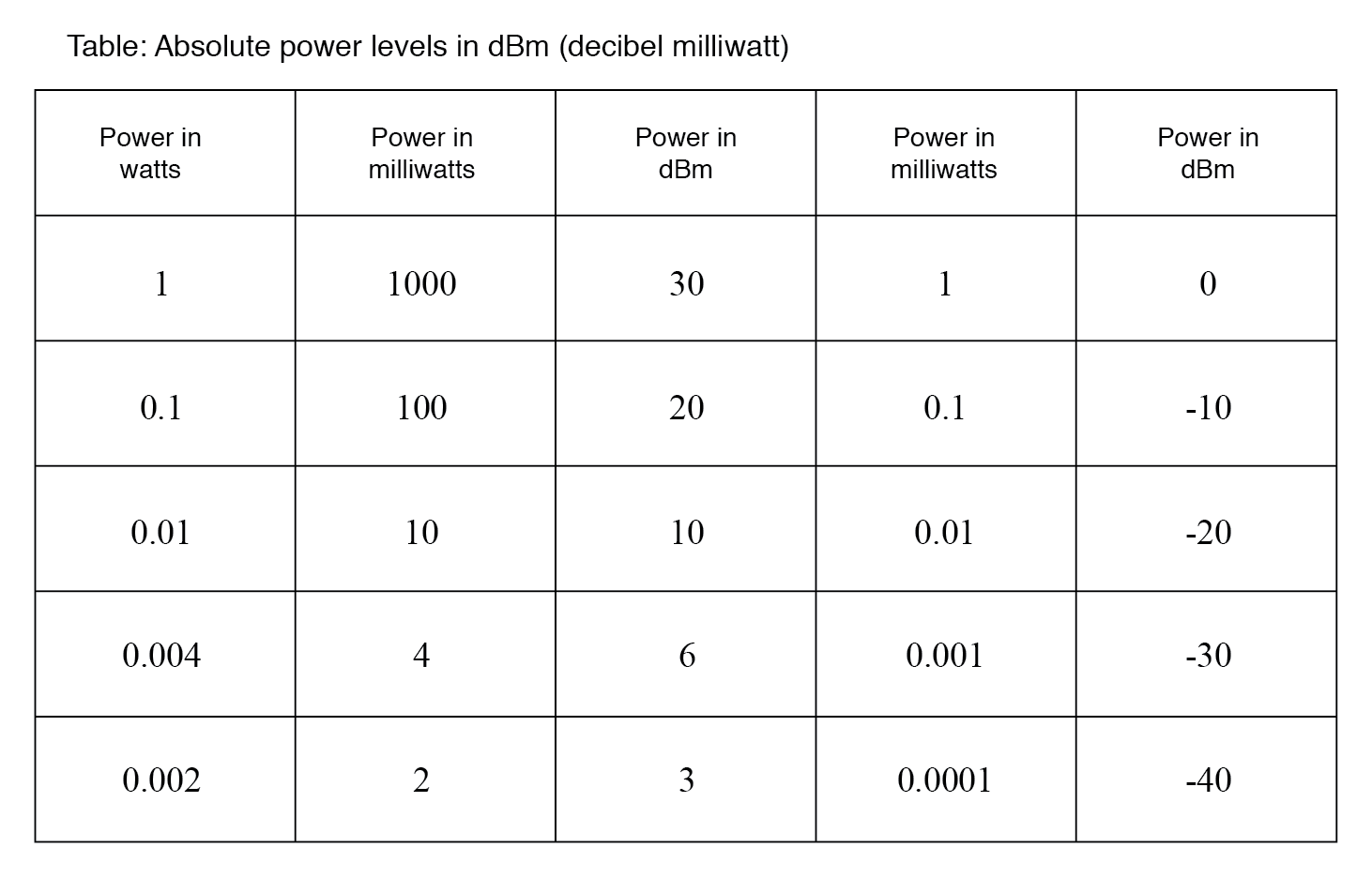# Absolute dB scales

## Chapter 1 - Amplifiers and Active Devices

### Decibel as Absolute Unit of Power

It is also possible to use the decibel as a unit of absolute power, in addition to using it as an expression of power gain or loss. A common example of this is the use of decibels as a measurement of sound pressure intensity. In cases like these, the measurement is made in reference to some standardized power level defined as 0 dB. For measurements of sound pressure, 0 dB is loosely defined as the lower threshold of human hearing, objectively quantified as 1 picowatt of sound power per square meter of area.

A sound measuring 40 dB on the decibel sound scale would be 104 times greater than the threshold of hearing. A 100 dB sound would be 1010 (ten billion) times greater than the threshold of hearing.

### Variations of Decibel Scales

Because the human ear is not equally sensitive to all frequencies of sound, variations of the decibel sound-power scale have been developed to represent physiologically equivalent sound intensities at different frequencies. Some sound intensity instruments were equipped with filter networks to give disproportionate indications across the frequency scale, the intent of which to better represent the effects of sound on the human body.

Three filtered scales became commonly known as the “A,” “B,” and “C” weighted scales. Decibel sound intensity indications measured through these respective filtering networks were given in units of dBA, dBB, and dBC. Today, the “A-weighted scale” is most commonly used for expressing the equivalent physiological impact on the human body, and is especially useful for rating dangerously loud noise sources.

### Decibel-milliwatts(dBm)

Another standard-referenced system of power measurement in the unit of decibels has been established for use in telecommunications systems. This is called the dBm scale. (figure below) The reference point, 0 dBm, is defined as 1 milliwatt of electrical power dissipated by a 600 Ω load. According to this scale, 10 dBm is equal to 10 times the reference power, or 10 milliwatts; 20 dBm is equal to 100 times the reference power, or 100 milliwatts. Some AC voltmeters come equipped with a dBm range or scale (sometimes labeled “DB”) intended for use in measuring AC signal power across a 600 Ω load. 0 dBm on this scale is, of course, elevated above zero because it represents something greater than 0 (actually, it represents 0.7746 volts across a 600 Ω load, voltage being equal to the square root of power times resistance; the square root of 0.001 multiplied by 600). When viewed on the face of an analog meter movement, this dBm scale appears compressed on the left side and expanded on the right in a manner not unlike a resistance scale, owing to its logarithmic nature.

Radiofrequency power measurements for low-level signals encountered in radio receivers use dBm measurements referenced to a 50 Ω load. Signal generators for the evaluation of radio receivers may output an adjustable dBm rated signal. The signal level is selected by a device called an attenuator, described in the next section.Absolute power levels in dBm (decibels referenced to 1 milliwatt).

### VU Scale

An adaptation of the dBm scale for audio signal strength is used in studio recording and broadcast engineering for standardizing volume levels, and is called the VU scale. VU meters are frequently seen on electronic recording instruments to indicate whether or not the recorded signal exceeds the maximum signal level limit of the device, where significant distortion will occur. This “volume indicator” scale is calibrated in according to the dBm scale, but does not directly indicate dBm for any signal other than steady sine-wave tones. The proper unit of measurement for a VU meter is volume units.

### Other Absolute dB Scales

When relatively large signals are dealt with, and an absolute dB scale would be useful for representing signal level, specialized decibel scales are sometimes used with reference points greater than the 1 mW used in dBm. Such is the case for the dBW scale, with a reference point of 0 dBW established at 1 Watt. Another absolute measure of power called the dBk scale references 0 dBk at 1 kW, or 1000 Watts.

REVIEW:

• The unit of the bel or decibel may also be used to represent an absolute measurement of power rather than just a relative gain or loss.
• For sound power measurements, 0 dB is defined as a standardized reference point of power equal to 1 picowatt per square meter.
• Another dB scale suited for sound intensity measurements is normalized to the same physiological effects as a 1000 Hz tone, and is called the dBA scale. In this system, 0 dBA is defined as any frequency sound having the same physiological equivalence as a 1 picowatt-per-square-meter tone at 1000 Hz.
• An electrical dB scale with an absolute reference point has been made for use in telecommunications systems. Called the dBm scale, its reference point of 0 dBm is defined as 1 milliwatt of AC signal power dissipated by a 600 Ω load.
• A VU meter reads audio signal level according to the dBm for sine-wave signals. Because its response to signals other than steady sine waves is not the same as true dBm, its unit of measurement is volume units.
• dB scales with greater absolute reference points than the dBm scale have been invented for high-power signals. The dBW scale has its reference point of 0 dBW defined as 1 Watt of power. The dBk scale sets 1 kW (1000 Watts) as the zero-point reference.

RELATED WORKSHEETS:

• Share
Published under the terms and conditions of the Design Science License